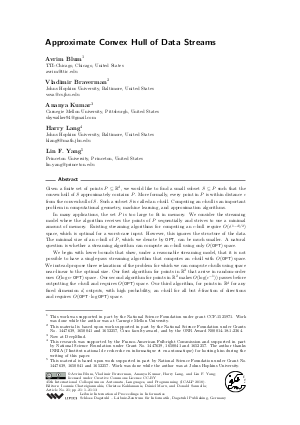Document# Approximate Convex Hull of Data Streams

### Authors Avrim Blum, Vladimir Braverman, Ananya Kumar, Harry Lang, Lin F. Yang## File

LIPIcs.ICALP.2018.21.pdf
• Filesize: 0.58 MB
• 13 pages

## Cite As

Avrim Blum, Vladimir Braverman, Ananya Kumar, Harry Lang, and Lin F. Yang. Approximate Convex Hull of Data Streams. In 45th International Colloquium on Automata, Languages, and Programming (ICALP 2018). Leibniz International Proceedings in Informatics (LIPIcs), Volume 107, pp. 21:1-21:13, Schloss Dagstuhl - Leibniz-Zentrum für Informatik (2018)
https://doi.org/10.4230/LIPIcs.ICALP.2018.21

## Abstract

Given a finite set of points P subseteq R^d, we would like to find a small subset S subseteq P such that the convex hull of S approximately contains P. More formally, every point in P is within distance epsilon from the convex hull of S. Such a subset S is called an epsilon-hull. Computing an epsilon-hull is an important problem in computational geometry, machine learning, and approximation algorithms. In many applications, the set P is too large to fit in memory. We consider the streaming model where the algorithm receives the points of P sequentially and strives to use a minimal amount of memory. Existing streaming algorithms for computing an epsilon-hull require O(epsilon^{(1-d)/2}) space, which is optimal for a worst-case input. However, this ignores the structure of the data. The minimal size of an epsilon-hull of P, which we denote by OPT, can be much smaller. A natural question is whether a streaming algorithm can compute an epsilon-hull using only O(OPT) space. We begin with lower bounds that show, under a reasonable streaming model, that it is not possible to have a single-pass streaming algorithm that computes an epsilon-hull with O(OPT) space. We instead propose three relaxations of the problem for which we can compute epsilon-hulls using space near-linear to the optimal size. Our first algorithm for points in R^2 that arrive in random-order uses O(log n * OPT) space. Our second algorithm for points in R^2 makes O(log(epsilon^{-1})) passes before outputting the epsilon-hull and requires O(OPT) space. Our third algorithm, for points in R^d for any fixed dimension d, outputs, with high probability, an epsilon-hull for all but delta-fraction of directions and requires O(OPT * log OPT) space.

## Subject Classification

##### ACM Subject Classification
• Theory of computation → Computational geometry
• Theory of computation → Sketching and sampling
• Theory of computation → Streaming models
##### Keywords
• Convex Hulls
• Streaming Algorithms
• Epsilon Kernels
• Sparse Coding

## Metrics

• Access Statistics
• Total Accesses (updated on a weekly basis)
0

## References

1. Pankaj K. Agarwal, Sariel Har-Peled, and Kasturi R. Varadarajan. Approximating extent measures of points. J. ACM, 51(4):606-635, 2004. URL: http://dx.doi.org/10.1145/1008731.1008736.
2. Pankaj K. Agarwal, Sariel Har-Peled, and Kasturi R. Varadarajan. Geometric approximation via coresets, pages 1-30. University Press, 2005.3. Pankaj K Agarwal and Hai Yu. A space-optimal data-stream algorithm for coresets in the plane. In Proceedings of the twenty-third annual symposium on Computational geometry, pages 1-10. ACM, 2007.4. Sunil Arya and Timothy M. Chan. Better epsilon-dependencies for offline approximate nearest neighbor search, euclidean minimum spanning trees, and epsilon-kernels. In Proceedings of the Thirtieth Annual Symposium on Computational Geometry, SOCG'14, pages 416:416-416:425, New York, NY, USA, 2014. ACM. URL: http://dx.doi.org/10.1145/2582112.2582161.
5. Jon Louis Bentley, Franco P. Preparata, and Mark G. Faust. Approximation algorithms for convex hulls. Commun. ACM, 25(1):64-68, jan 1982. URL: http://dx.doi.org/10.1145/358315.358392.
6. David M Blei. Probabilistic topic models. Communications of the ACM, 55(4):77-84, 2012.7. Avrim Blum, Sariel Har-Peled, and Benjamin Raichel. Sparse approximation via generating point sets. In Proceedings of the Twenty-Seventh Annual ACM-SIAM Symposium on Discrete Algorithms, pages 548-557, 2016.8. Timothy M. Chan. Faster core-set constructions and data-stream algorithms in fixed dimensions. Computational Geometry, 35(1):20-35, 2006. URL: http://dx.doi.org/10.1016/j.comgeo.2005.10.002.
9. Timothy M. Chan. Dynamic streaming algorithms for epsilon-kernels. In Proc. 32nd Annu. Sympos. Comput. Geom. (SoCG), 2016.10. Timothy M. Chan. Applications of chebyshev polynomials to low-dimensional computational geometry. In Proc. 33rd Annu. Sympos. Comput. Geom. (SoCG), 2017.11. John Hershberger and Subhash Suri. Adaptive sampling for geometric problems over data streams. In Proceedings of the Twenty-third ACM SIGMOD-SIGACT-SIGART Symposium on Principles of Database Systems, PODS '04, pages 252-262, New York, NY, USA, 2004. ACM. URL: http://dx.doi.org/10.1145/1055558.1055595.
12. David M. Mount Sunil Arya, Guilherme D. da Fonseca. Near-optimal epsilon-kernel construction and related problems. In Proc. 33rd Annu. Sympos. Comput. Geom. (SoCG), 2017.13. Hamid Zarrabi-Zadeh. An almost space-optimal streaming algorithm for coresets in fixed dimensions. Algorithmica, 60(1):46-59, 2011. URL: http://dx.doi.org/10.1007/s00453-010-9392-2.
X

Feedback for Dagstuhl Publishing